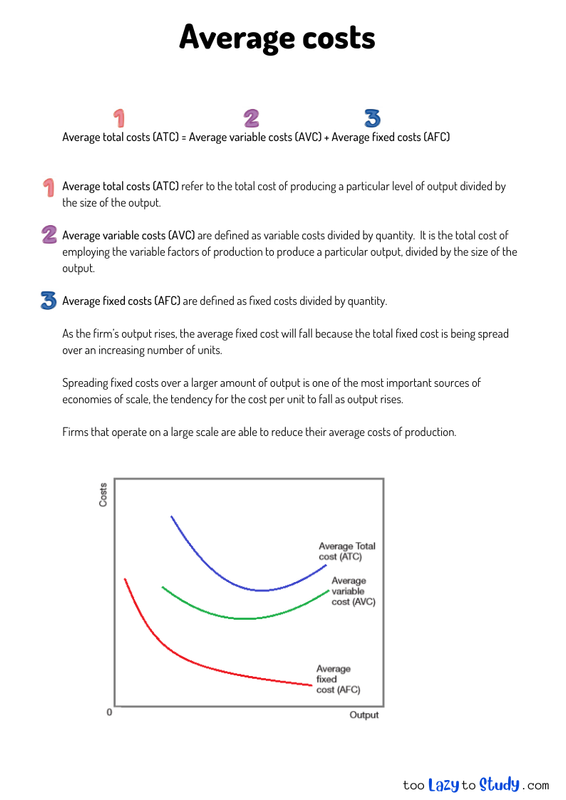top of page

Overview

Average total costs (ATC) = Average variable costs (AVC) + Average fixed costs (AFC)

Average total costs (ATC) refer to the total cost of producing a particular level of output divided by the size of the output.

Average variable costs (AVC) are defined as variable costs divided by quantity. It is the total cost of employing the variable factors of production to produce a particular output, divided by the size of the output.

Average fixed costs (AFC) are defined as fixed costs divided by quantity.

As the firm’s output rises, the average fixed cost will fall because the total fixed cost is being spread over an increasing number of units.

Spreading fixed costs over a larger amount of output is one of the most important sources of economies of scale, the tendency for the cost per unit to fall as output rises.

Firms that operate on a large scale are able to reduce their average costs of production.# Average costs

#### Perfect for A level, GCSEs and O levels!

👑Subscribe to the Economics Study Pack and Download economics notes in PDF and EDITABLE versions!bottom of page